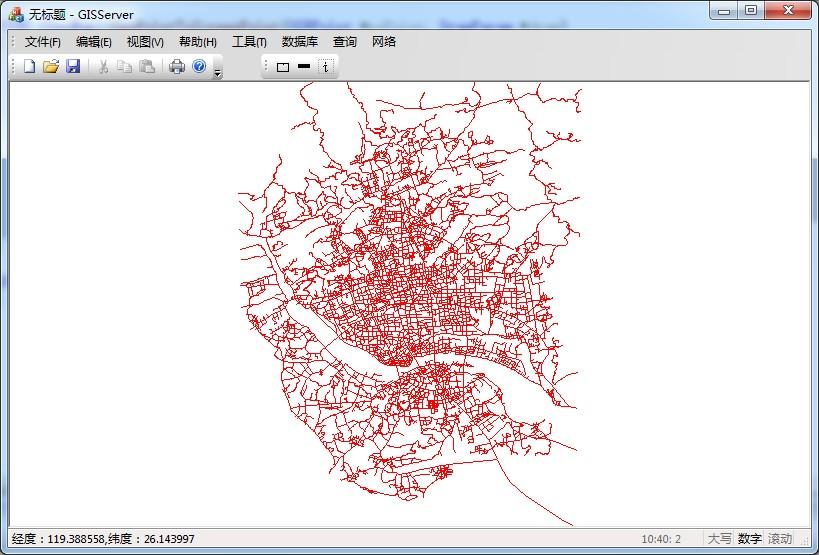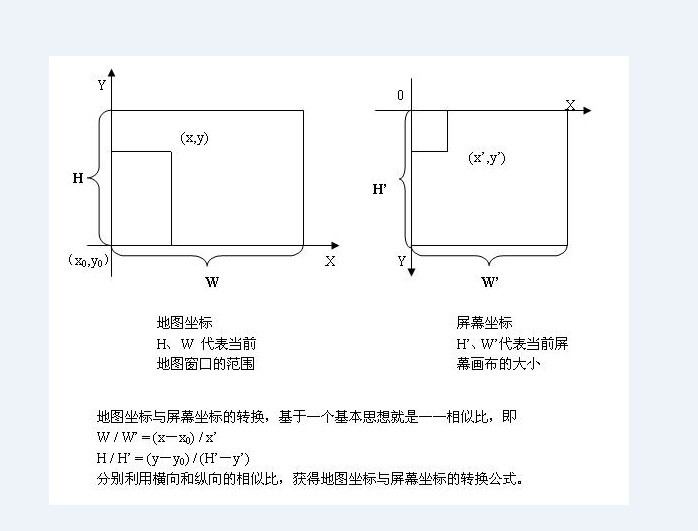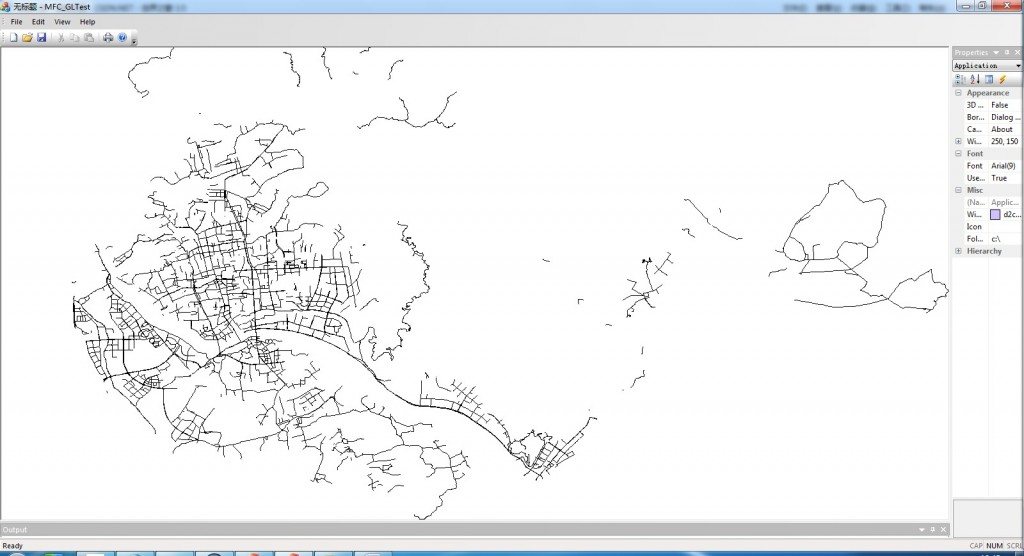地理坐标与屏幕坐标转换（VC++）

年薪达不到25W大数据工程师、拿不到Offer全额退款->>>OGRPoint ptCenter = GetMapCenter();		//地图中心点
POINT pt;
pt.x = draw->m_ScreenWidth/2 + (long)((poPoint->getX()-ptCenter.getX())*draw->m_Scale+0.5);
pt.y = draw->m_ScreenHeigh/2 - (long)((poPoint->getY()-ptCenter.getY())*draw->m_Scale+0.5);
return pt;

OGRPoint ptCenter = GetMapCenter();		//地图中心点
POINT pt;
pt.x = draw->m_ScreenWidth/2 + (long)((poPoint->getX()-ptCenter.getX())*draw->m_Scale+0.5);
pt.y = draw->m_ScreenHeigh/2 - (long)((poPoint->getY()-ptCenter.getY())*draw->m_Scale+0.5);
return pt;double xScale = rect.Width()/width;		//x方向的比例系数
double yScale = rect.Height()/height;	//y方向的比例系数
double scale = max(xScale,yScale);

pts[index].x = (int)((poPoint->getX()-minx)*scale);
pts[index].y = (int)(rect.Height()-(poPoint->getY()-miny)*scale);

GDI设备描述表（device context,DC）包含了特定的显示设备的信息、图像的显示属性及特定的显示窗口等信息，用GDI编程的第一步必须获取当前的设备描述表的句柄（hDC），然后将该句柄作为参数调用GDI提供的API函数。我们在绘图时，通常是各个地理实体分别绘制，以线串为例：

//以下代码是根据直线的方法绘制
for (int index = 0; index < numPoints - 1; index ++)
{
dcMem.MoveTo(pts[index]);
dcMem.LineTo(pts[index + 1]);
}